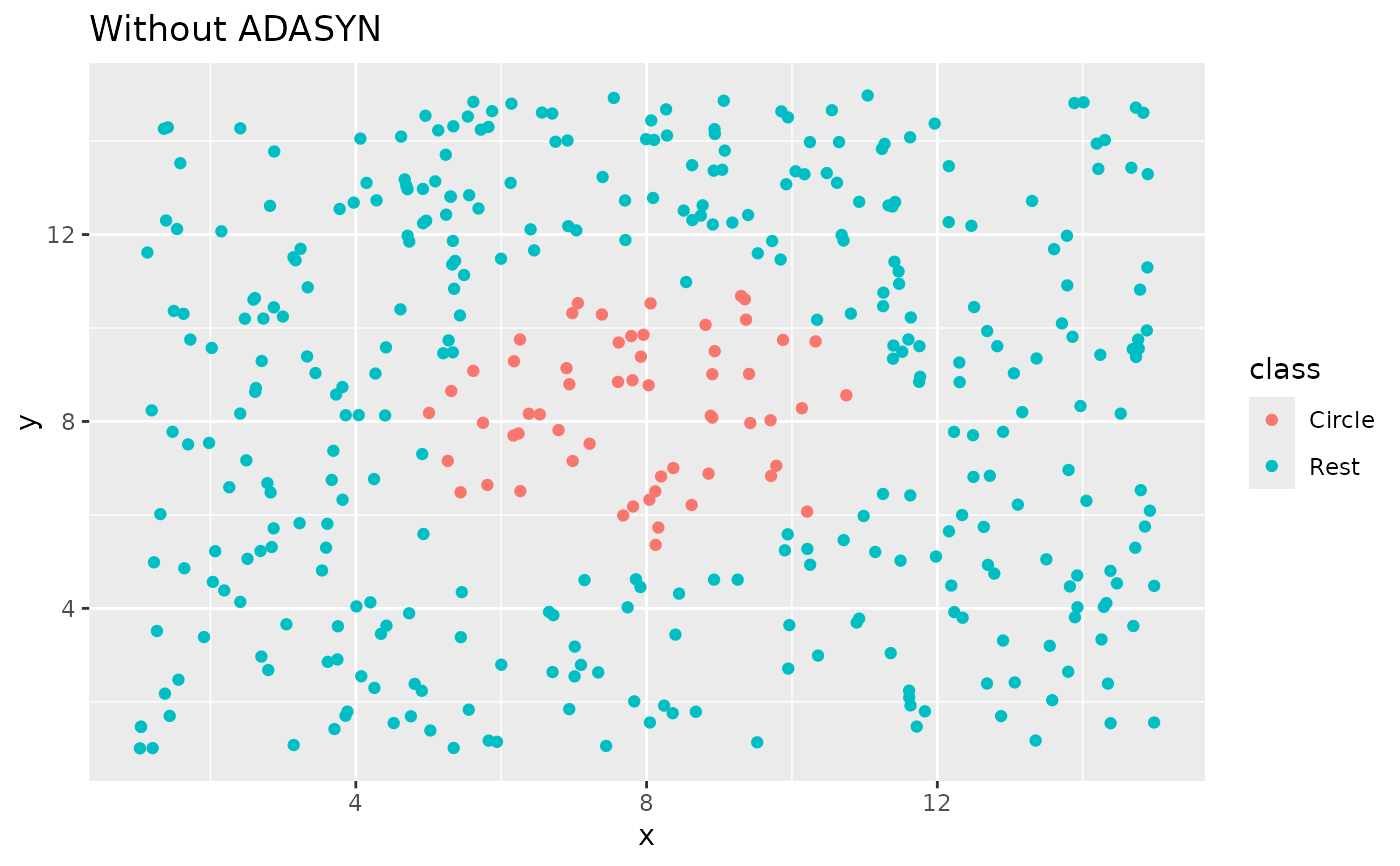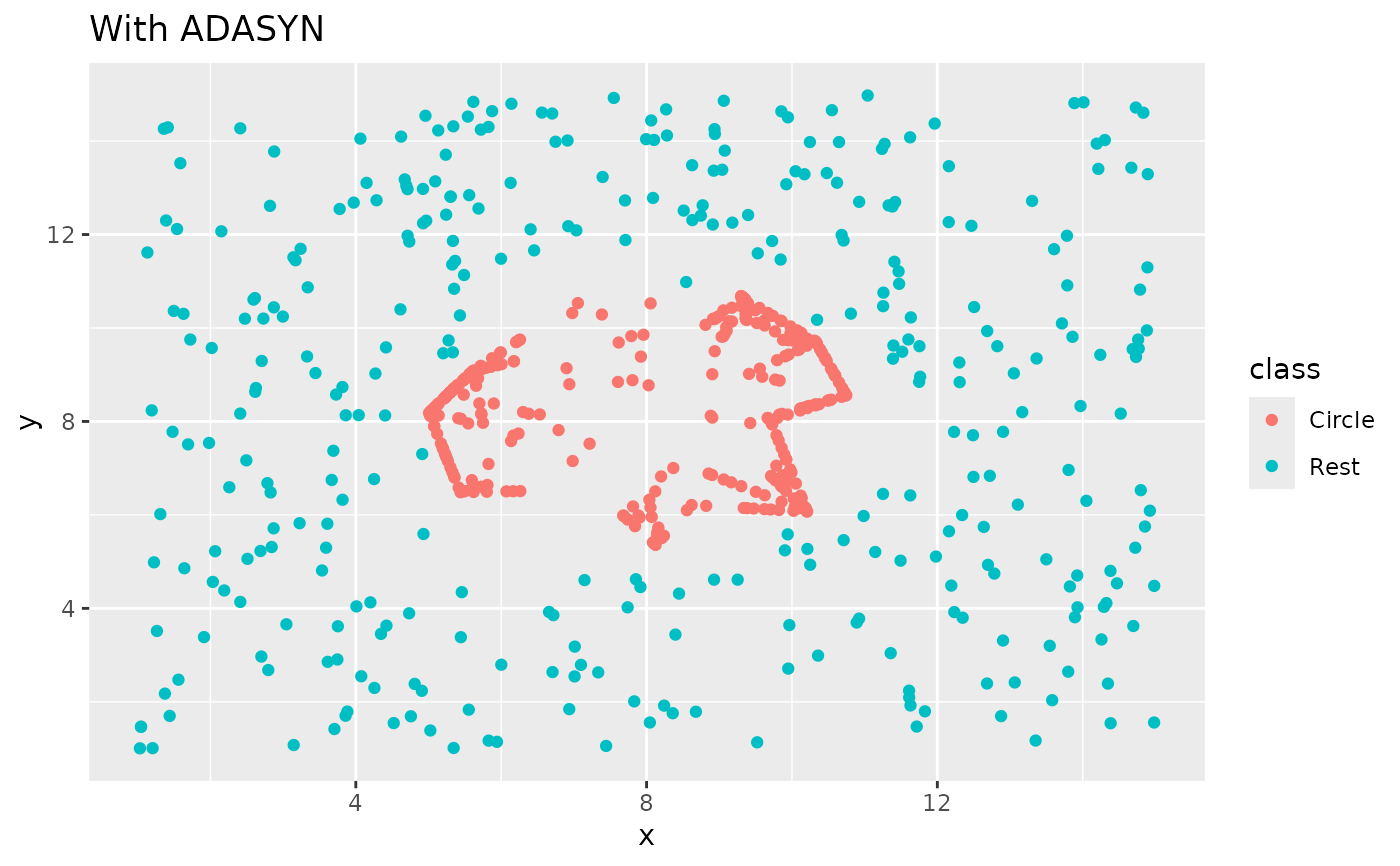step_adasyn creates a specification of a recipe step that generates synthetic positive instances using ADASYN algorithm.

step_adasyn(
recipe,
...,
role = NA,
trained = FALSE,
column = NULL,
over_ratio = 1,
neighbors = 5,
skip = TRUE,
seed = sample.int(10^5, 1),
)

tidy(x, ...)

## Arguments

recipe A recipe object. The step will be added to the sequence of operations for this recipe. One or more selector functions to choose which variable is used to sample the data. See selections() for more details. The selection should result in single factor variable. For the tidy method, these are not currently used. Not used by this step since no new variables are created. A logical to indicate if the quantities for preprocessing have been estimated. A character string of the variable name that will be populated (eventually) by the ... selectors. A numeric value for the ratio of the majority-to-minority frequencies. The default value (1) means that all other levels are sampled up to have the same frequency as the most occurring level. A value of 0.5 would mean that the minority levels will have (at most) (approximately) half as many rows than the majority level. An integer. Number of nearest neighbor that are used to generate the new examples of the minority class. A logical. Should the step be skipped when the recipe is baked by bake.recipe()? While all operations are baked when prep.recipe() is run, some operations may not be able to be conducted on new data (e.g. processing the outcome variable(s)). Care should be taken when using skip = TRUE as it may affect the computations for subsequent operations An integer that will be used as the seed when applied. A character string that is unique to this step to identify it. A step_adasyn object.

## Value

An updated version of recipe with the new step added to the sequence of existing steps (if any). For the tidy method, a tibble with columns terms which is the variable used to sample.

## Details

All columns in the data are sampled and returned by juice() and bake().

All columns used in this step must be numeric with no missing data.

When used in modeling, users should strongly consider using the option skip = TRUE so that the extra sampling is not conducted outside of the training set.

He, H., Bai, Y., Garcia, E. and Li, S. 2008. ADASYN: Adaptive synthetic sampling approach for imbalanced learning. Proceedings of IJCNN 2008. (IEEE World Congress on Computational Intelligence). IEEE International Joint Conference. pp.1322-1328.

## Examples

library(recipes)
library(modeldata)
data(okc)

sort(table(okc$Class, useNA = "always")) #> #> <NA> stem other #> 0 9539 50316 ds_rec <- recipe(Class ~ age + height, data = okc) %>% step_meanimpute(all_predictors()) %>% step_adasyn(Class) %>% prep() sort(table(bake(ds_rec, new_data = NULL)$Class, useNA = "always"))
#>
#>  <NA>  stem other
#>     0 50316 50316
# since skip defaults to TRUE, baking the step has no effect
baked_okc <- bake(ds_rec, new_data = okc)
table(baked_okc\$Class, useNA = "always")
#>
#>  stem other  <NA>
#>  9539 50316     0
library(ggplot2)

ggplot(circle_example, aes(x, y, color = class)) +
geom_point() +